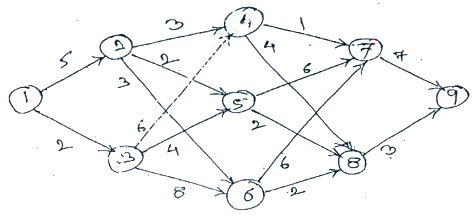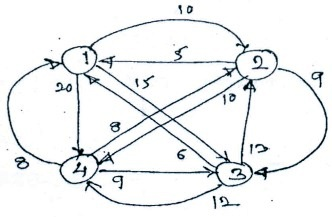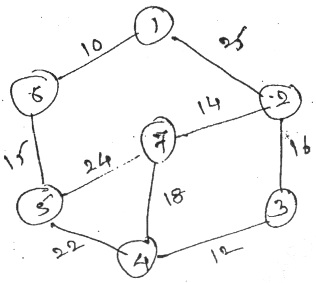MORE IN Analysis of Algorithms
MU Computer Engineering (Semester 4)
Analysis of Algorithms
December 2014
Total marks: --
Total time: --
INSTRUCTIONS
(1) Assume appropriate data and state your reasons
(2) Marks are given to the right of every question
(3) Draw neat diagrams wherever necessary

1 (a) Write an algorithm to find minimum and maximum value using divide and conquer and also drive its complexity.
10 M
1 (b) To sort the given set of number using insertion sort and also show the result of each pass.
<11, 7, 17, 3, 9, 29, 85, 9>
10 M

2 (a) Find an optional solution to the knapsack instance n=7 m=15, Profit = {10, 5, 15, 7, 6, 18, 3}
Weight = {2, 3, 5, 7, 1, 4, 1}
10 M
2 (b) Explain optimal storage on tape with example.
10 M

3 (a) Find a minimum cost path from 1 to 9 in the given graph using dynamic programming.10 M
3 (b) Find the path of travelling sales person problem of given graph.10 M

4 (a) To generate the Huffman code for given set of frequencies.
1, 1, 2, 3, 4, 8, 13, 21
10 M
4 (b) To implement the Knuth-Morris-Pratt, string matching algorithm.
10 M

5 (a) To find MST of following graph using Prim's and Kruskal's algorithm.10 M
5 (b) Explain flow shop scheduling using suitable data.
10 M

Write notes on: (any two): -
6 (a) N-Queen problem.
10 M
6 (b) Randomized Algorithm
10 M
6 (c) Tries
10 M
6 (d) The 15 Puzzle problem.
10 M

More question papers from Analysis of Algorithms